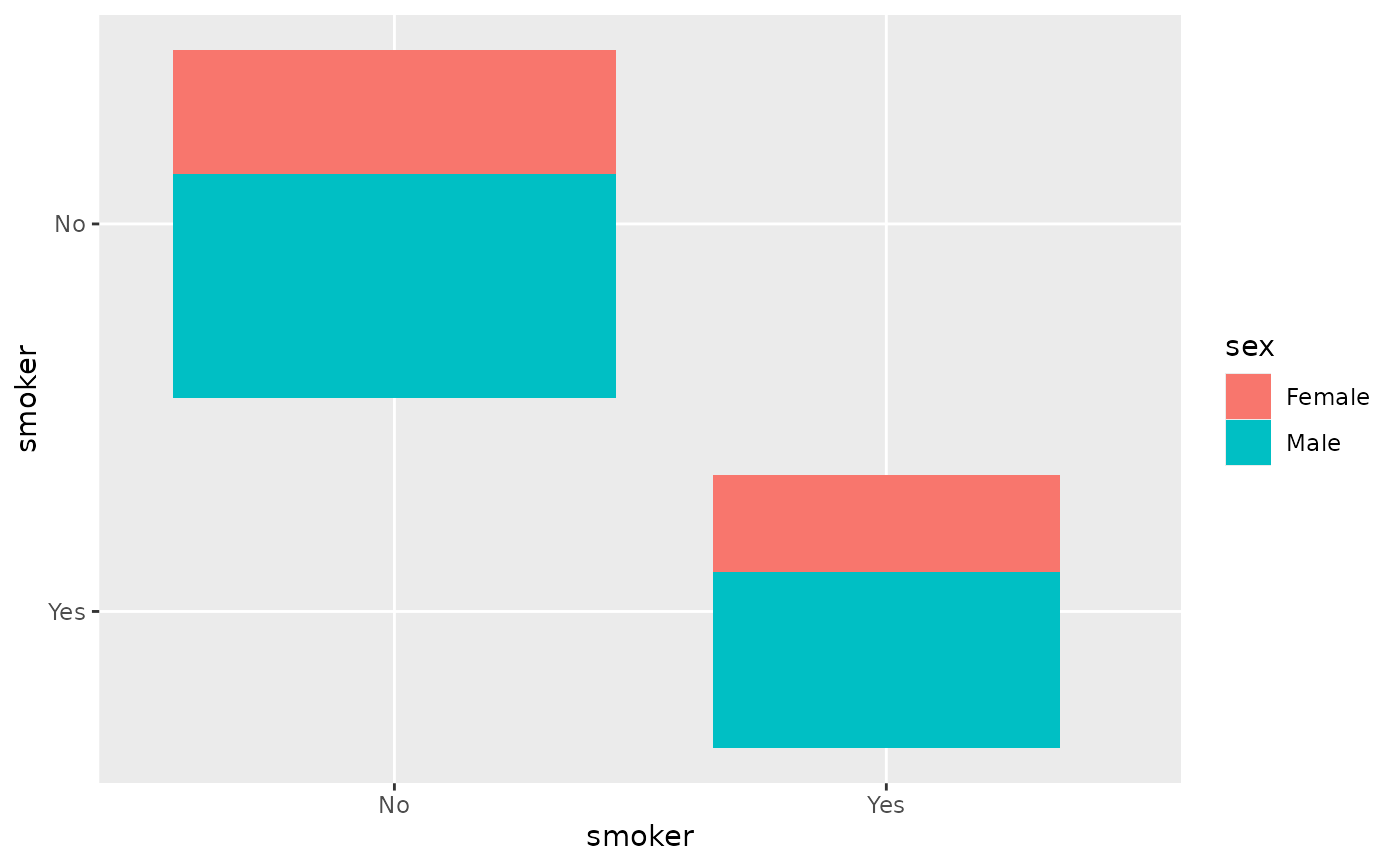Plot the number of observations by using rectangles with proportional areas.

ggally_count(data, mapping, ...)

ggally_countDiag(data, mapping, ...)

## Arguments

data data set using aesthetics being used other arguments passed to geom_tile(...)

## Details

You can adjust the size of rectangles with the x.width argument.

## Author

Joseph Larmarange

## Examples

# Small function to display plots only if it's interactive
p_ <- GGally::print_if_interactive

data(tips, package = "reshape")
p_(ggally_count(tips, mapping = ggplot2::aes(x = smoker, y = sex)))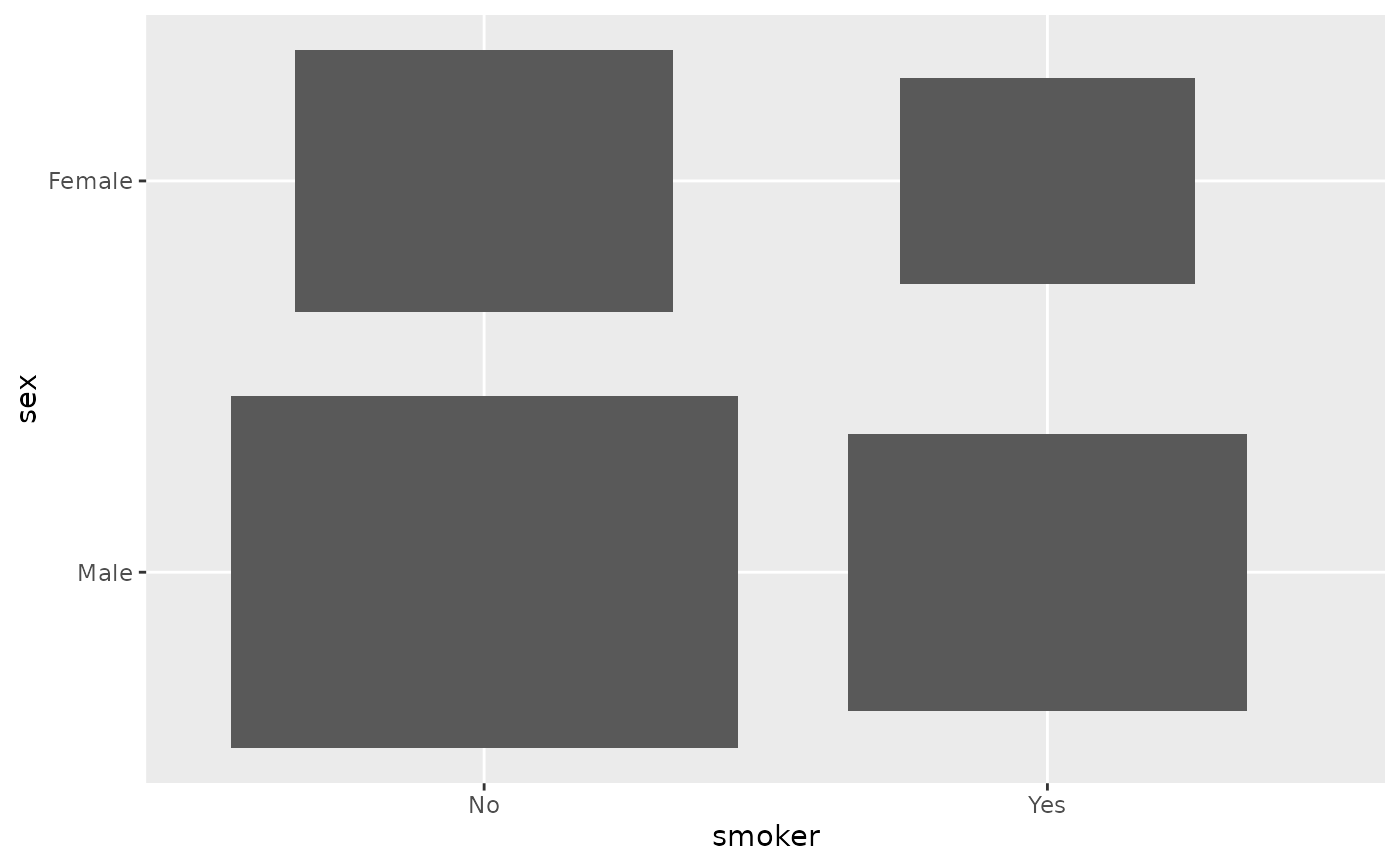p_(ggally_count(tips, mapping = ggplot2::aes(x = smoker, y = sex, fill = day)))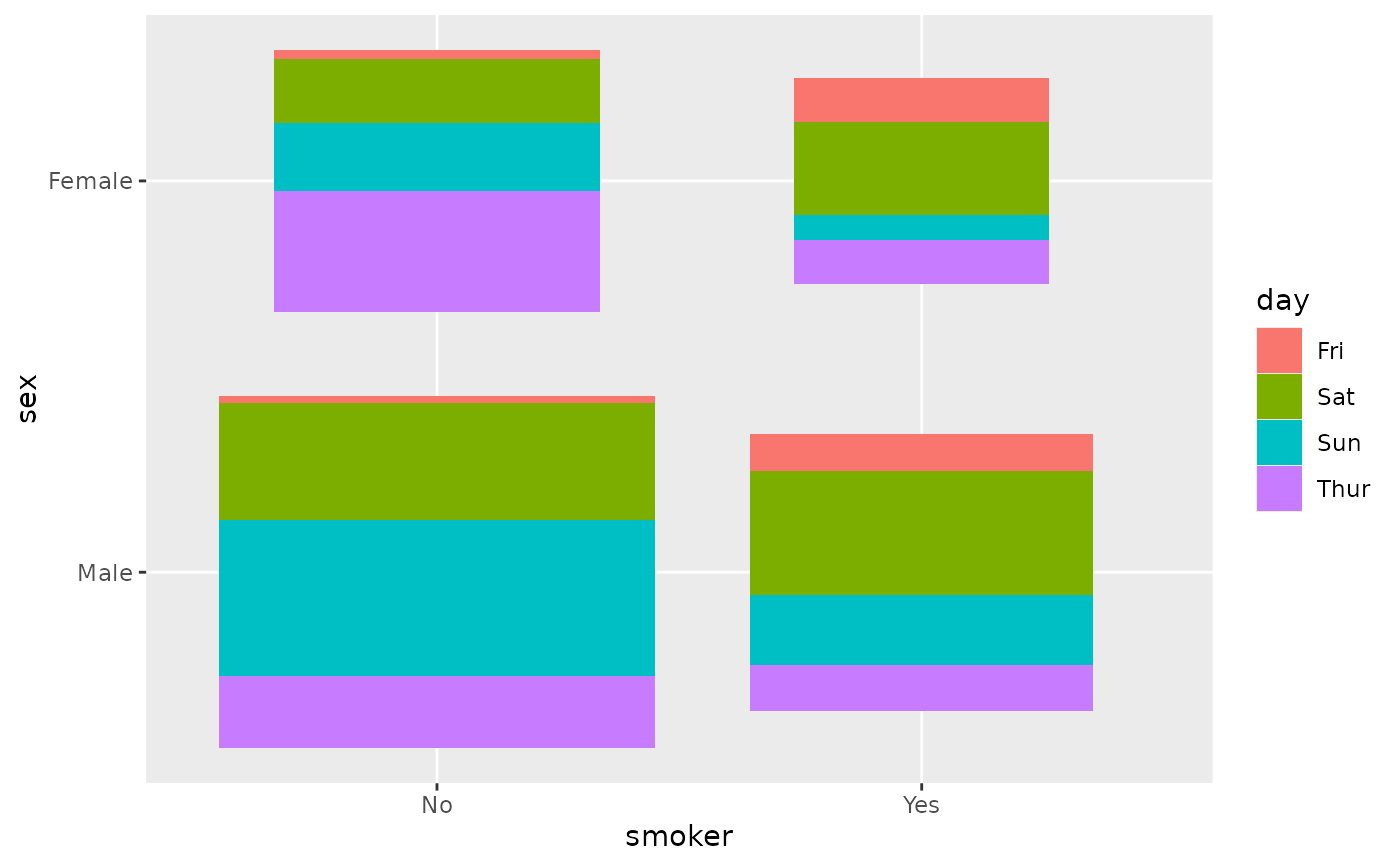p_(ggally_count(
as.data.frame(Titanic),
mapping = ggplot2::aes(x = Class, y = Survived, weight = Freq)
))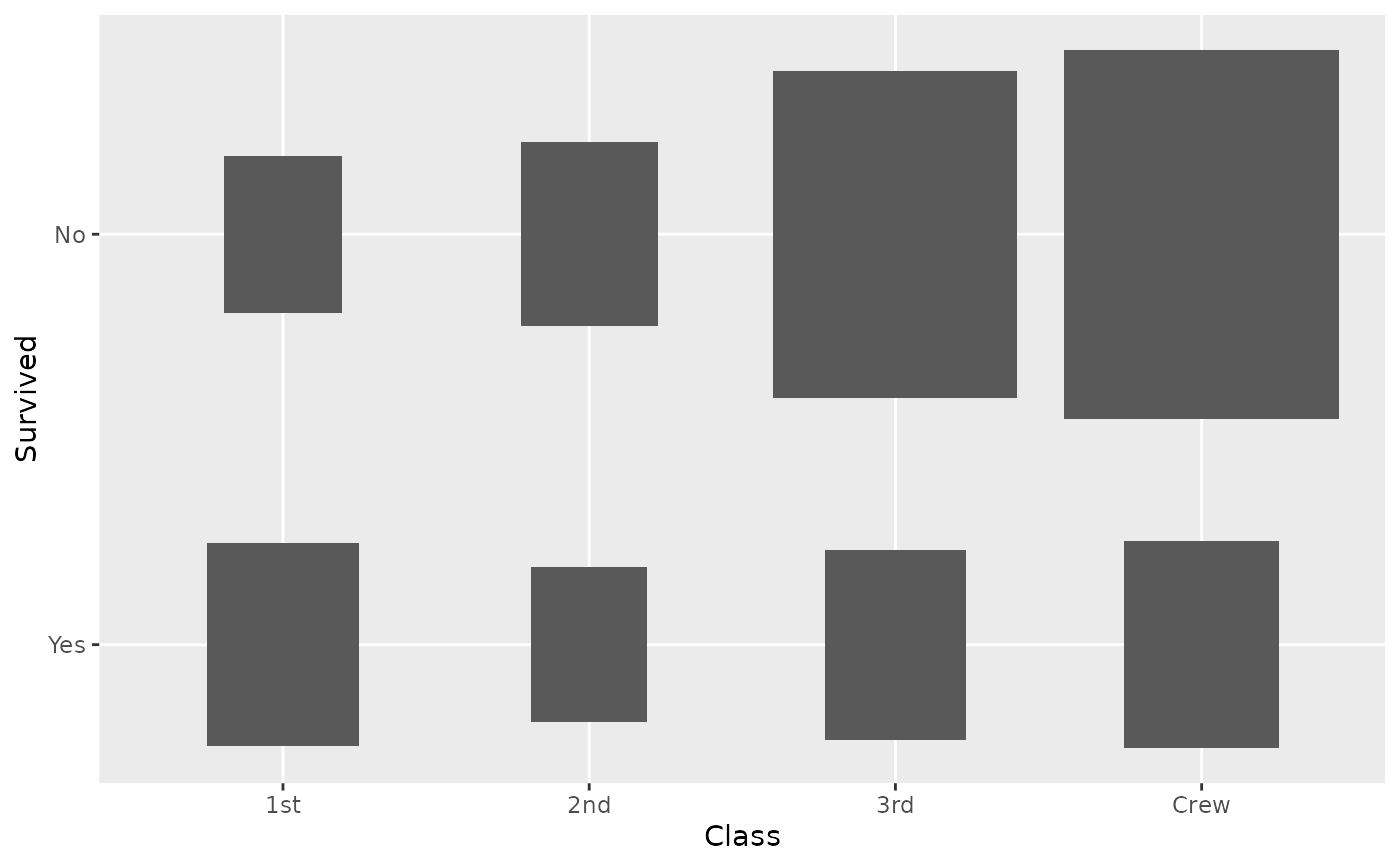p_(ggally_count(
as.data.frame(Titanic),
mapping = ggplot2::aes(x = Class, y = Survived, weight = Freq),
x.width = 0.5
))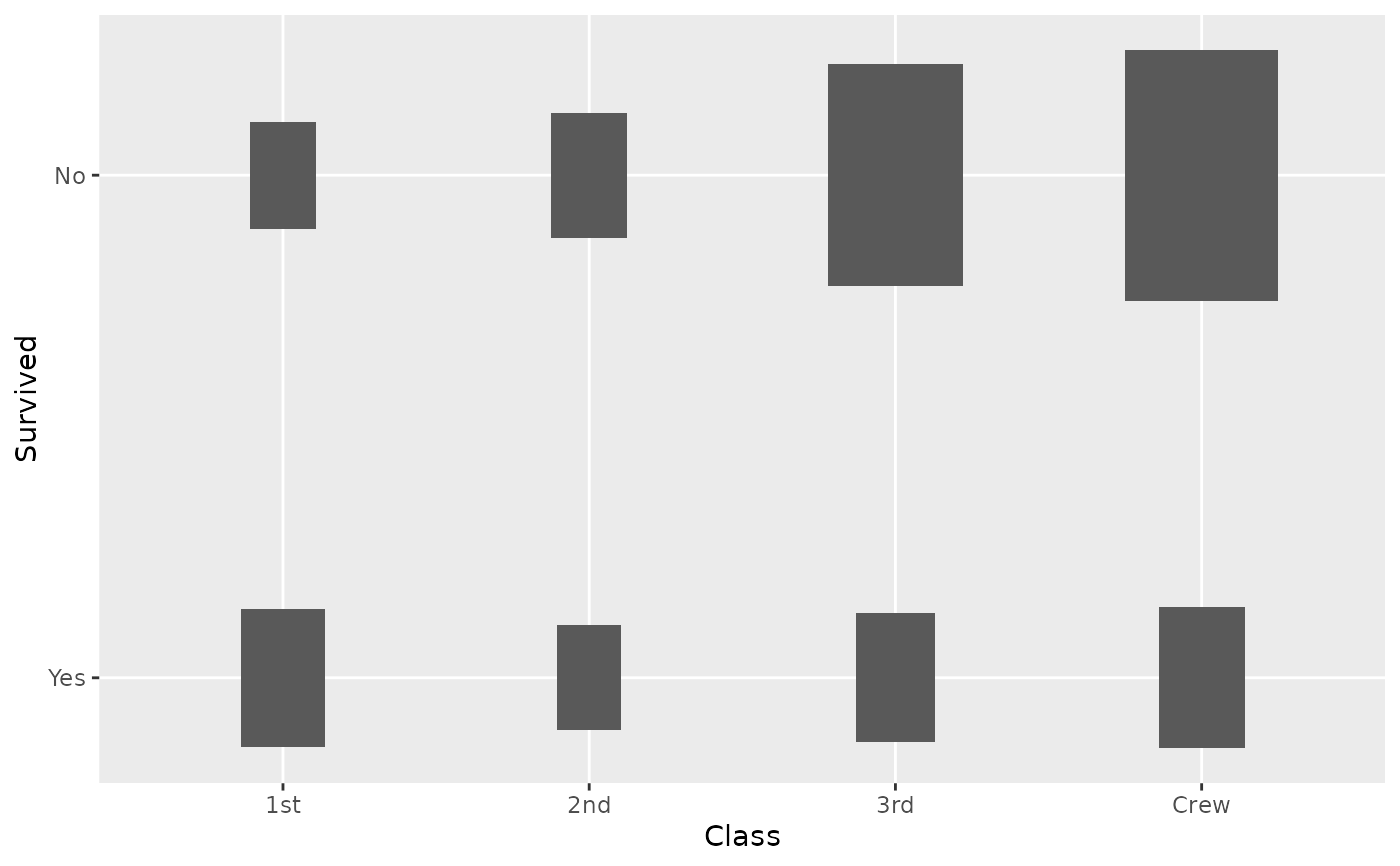# Small function to display plots only if it's interactive
p_ <- GGally::print_if_interactive

p_(ggally_countDiag(tips, mapping = ggplot2::aes(x = smoker)))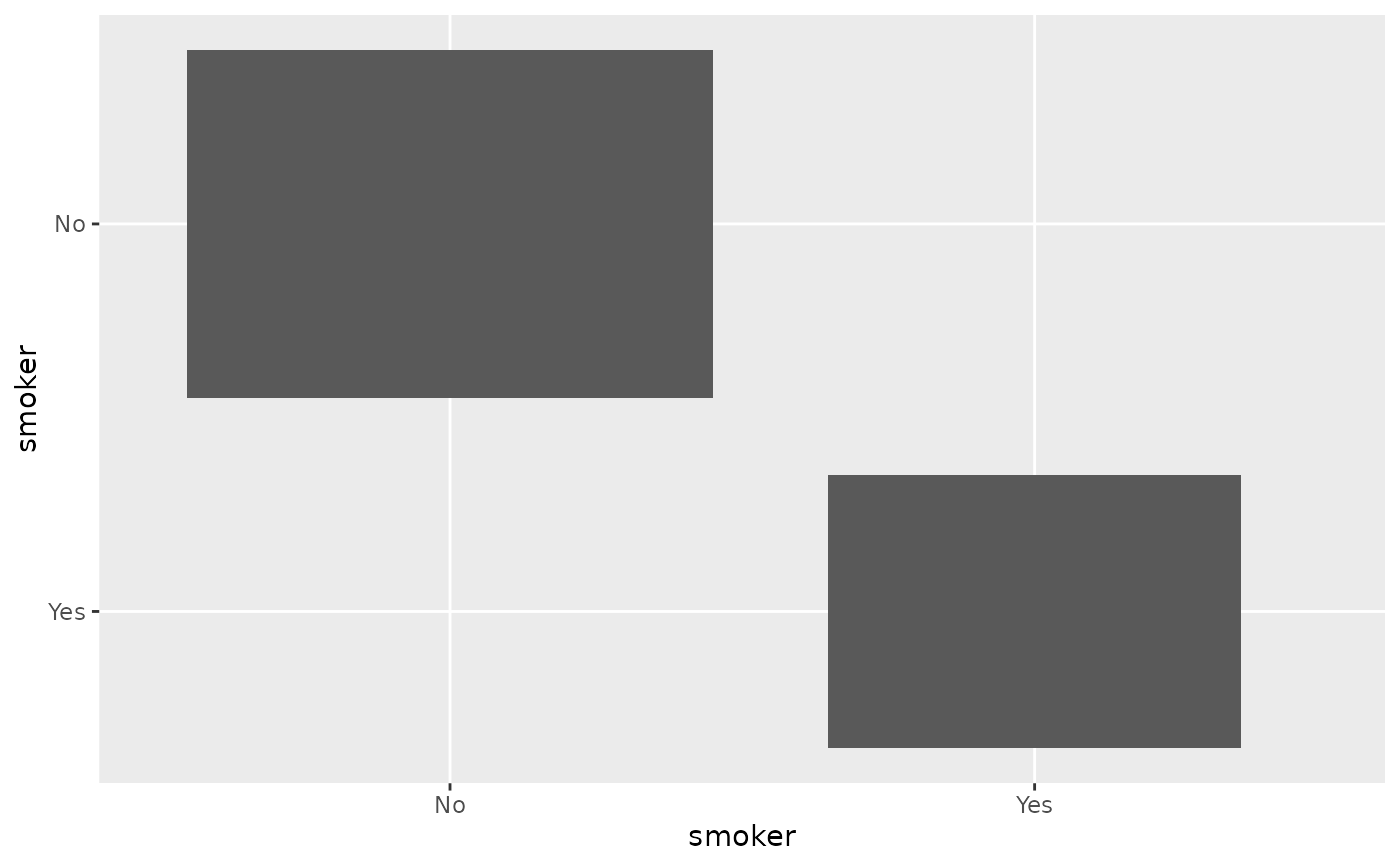p_(ggally_countDiag(tips, mapping = ggplot2::aes(x = smoker, fill = sex)))